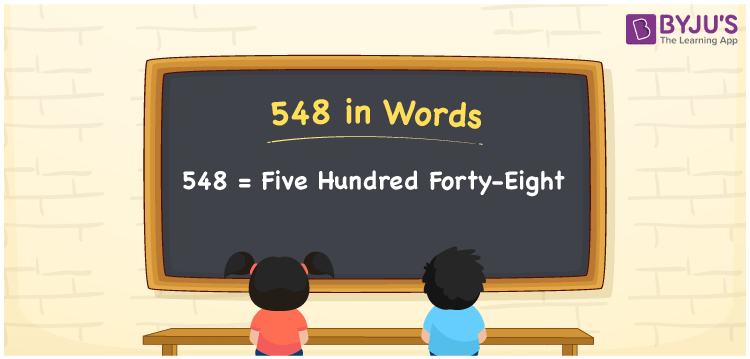# 548 in words

548 in words is written as Five Hundred and Forty Eight. 548 represents the count or value. The article on Counting Numbers can give you an idea about count or counting. The number 548 is used in expressions that relate to money, distance, length, year and others. Let us consider an example for 548. ”The shrine on a hilltop had Five Hundred and Forty Eight steps.”

 548 in words Five Hundred and Forty Eight Five Hundred and Forty Eight in Numbers 548

## 548 in English Words## How to Write 548 in Words?

We can convert 548 to words using a place value chart. The number 548 has 3 digits, so let’s make a chart that shows the place value up to 3 digits.

 Hundreds Tens Ones 5 4 8

Thus, we can write the expanded form as:

= 5 × Hundred + 4 × Ten + 8 × One

= 5 × 100 + 4 × 10 + 8 × 1

= 548

= Five Hundred and Forty Eight.

548 is the natural number that is succeeded by 547 and preceded by 549.

548 in words – Five Hundred and Forty Eight.

Is 548 an odd number? – No.

Is 548 an even number? – Yes.

Is 548 a perfect square number? – No.

Is 548 a perfect cube number? – No.

Is 548 a prime number? – No.

Is 548 a composite number? – Yes.

## Solved Example

1. Write the number 548 in expanded form

Solution: 5 × 100 + 4 × 10 + 8 × 1

We can write 548 = 500 + 40 + 8

= 5 × 100 + 4 × 10 + 8 × 1

## Frequently Asked Questions on 548 in words

Q1

### How to write 548 in words?

548 in words is written as Five Hundred and Forty Eight.
Q2

### Is 548 a perfect square number?

No. 548 is not a perfect square number.
Q3

### Is 548 a prime number?

No. 548 is not a prime number.